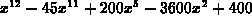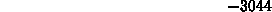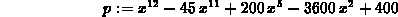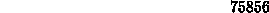Next: Choosing names for Up: Labels Previous: Labels

### What is a label?

In Maple, you can provide a name for the result of a Maple command. If you do this, then you can use the name you provided to refer to the result in other commands. For example, suppose you wanted to compute the value of the expressionfor several values of x. One way to do this in Maple is to simply use the subs command as follows.

```  > subs(x=1,x^12-45*x^11+200*x^5-3600*x^2+400);
``````  > subs(x=-2,x^12-45*x^11+200*x^5-3600*x^2+400);
```However, if you want the value of the expression for several values of x, then a more efficient way to do this is to label your expression and then use the label in the subs commands, as shown below.

```  > p := x^12-45*x^11+200*x^5-3600*x^2+400;
``````  > subs(x=1,p);
``````  > subs(x=-2,p);
```In the example above, the label was chosen to be the letter p. We could easily have chosen another letter, there is nothing special about the letter p. Also, labels don't have to consist of single letters - we could have used a sequence of several letters like poly (short for polynomial) for our label.

William W. Farr
Mon Aug 28 09:31:56 EDT 1995